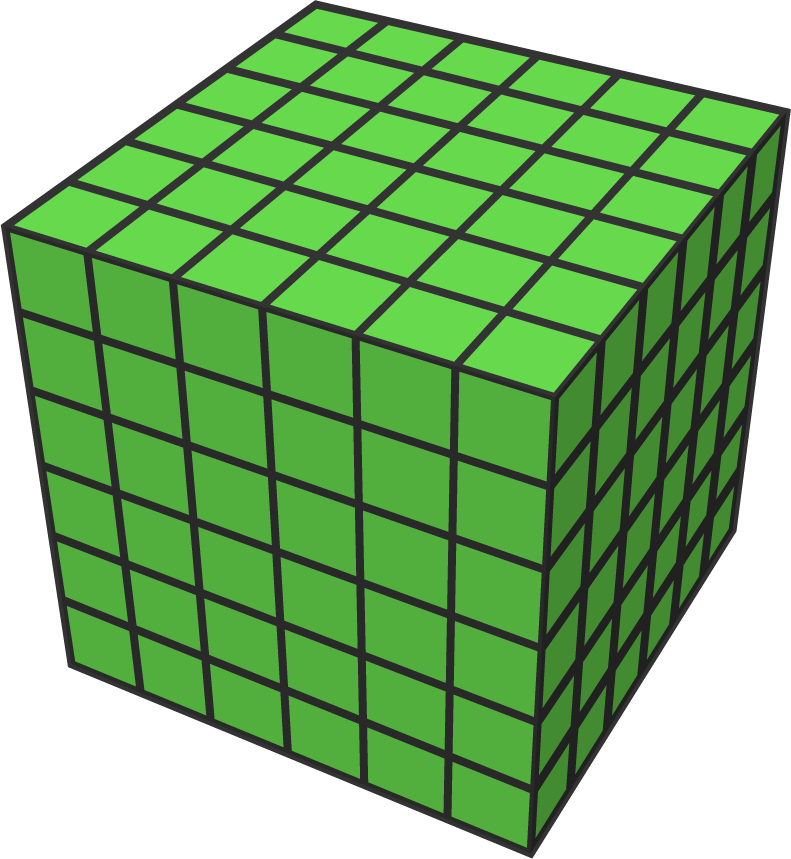# Visible cubes

Geometry Level 1Consider a $6\times 6\times 6$ cube made up of $216$ small cubes.

How many of these small $1\times 1\times 1$ cubes are visible by rotating the larger cube?

×